# Merge and Append Using Stata: How to Merge and Append Datasets

This guide discusses basic techniques to merge and append datasets using Stata.

## Merge

- We use merge  to add variables to a dataset.

- Merging two datasets requires that both have at least one variable in common (either string or numeric), which is also called the identifier variable.

- The common variables or the identifier variables must have the same name.

- If you merge string variables, make sure the categories have the same spelling (e.g., the spelling of the name of each country should be the same).

- Inspect each dataset carefully before merging. While merging two panel datasets, for example, look for two common variables: entity id (e.g., country, state) and time (e.g., year, month).

- This guide discusses different data merging techniques available in Stata. Note down some useful jargons I use throughout this guide:

master dataset: starting dataset/dataset in memory

using dataset: dataset you want to merge

matched dataset: dataset generated after the merge

master + using = matched

1. One-to-One Merge

We use one-to-one merge when the common variable(s) uniquely identifies each observation in each of the datasets. Let us merge the following two datasets, named mydata1 (master dataset) and mydata2 (using dataset).

mydata1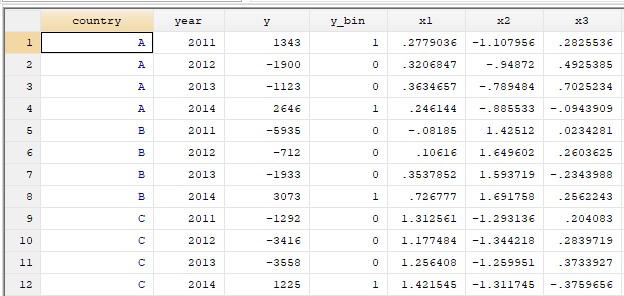mydata2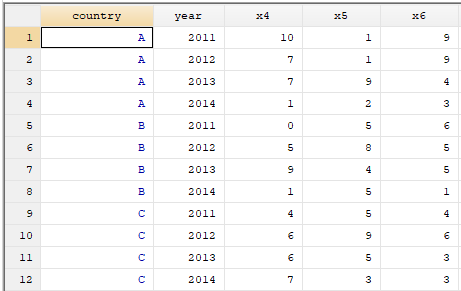Notice that the above datasets have two common variables: country and year. For merging these two datasets, we need to use one-to-one method because the combination of the values of country and year uniquely identifies observations in both datasets.

Use the following Stata commands for 1:1 merge.

###### Result                      Number of obs     -----------------------------------------     Not matched                             0     Matched                                12  (_merge==3)     -----------------------------------------

The resulting merged/matched dataset is as follows.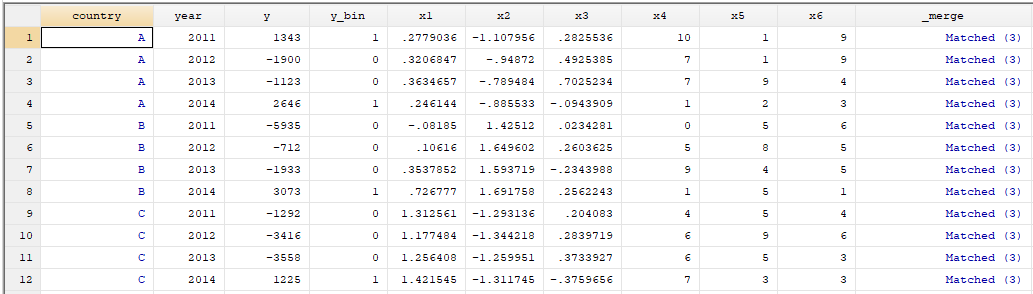Step-by-step procedure for your own data:

• When working on your own data, after the use command, write the path name of the location of your master dataset (in my case, mydata1 is located at "C:/Users/ma3482/Documents/Merge-Append/mydata1.dta"). So, I used the following codes:

use "C:/Users/ma3482/Documents/Merge-Append/mydata1.dta"

• Identify the common variables in your dataset. In my case, country and year are the common variables. Make sure that after the using command, you are writing the path name where your using dataset is located (in my case, mydata2 is located at "C:/Users/ma3482/Documents/Merge-Append/mydata2.dta"). I used the following codes:

merge 1:1 country year using "C:/Users/ma3482/Documents/Merge-Append/mydata2.dta"

Notes:

- 1:1 merge is less common than m:1 and 1:m merges.

- If you want to retain only selected variables from the "using" file, you need to use keepusing command and tell Stata which variables you want to keep. For example, if you want to merge mydata1 and mydata2, and want to merge variables x4 and x5 only from mydata2 to mydata1, use the following codes:

use https://dss.princeton.edu/training/mydata1.dta, clear

merge 1:1 country year using https://dss.princeton.edu/training/mydata2.dta, keepusing (x4 x5)

- In the merged/matched dataset above, notice that a new variable _merge has also been created because of merge. Usually, there are three possible values for this _merge  variable. The meaning of these values are as follows:

• _merge = 1: A case that is present in the master dataset only.
• _merge = 2: A case that is present in the using dataset only.
• _merge = 3: A case that is present in both datasets.

- If you want to keep only observations in both datasets, type:

use https://dss.princeton.edu/training/mydata1.dta, clear

merge 1:1 country year using https://dss.princeton.edu/training/mydata2.dta, keepusing (x4 x5)keep (match)

Note: You need to drop the _merge variable if you want to conduct more merging tasks using your master dataset. For dropping the variable type:

drop _merge

2. Many-to-One Merge

We use many-to-one merge when the common variable(s) uniquely identifies the observations in the using data, but not necessarily in the master data. Let us merge the following two datasets, named mydata1 (master dataset) and mydata3 (using dataset).

mydata1mydata3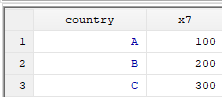Notice that country is the common variable in above two datasets.

Use the following Stata commands for m:1 merge.

###### Result                      Number of obs     -----------------------------------------     Not matched                             0     Matched                                12  (_merge==3)     -----------------------------------------

The resulting merged/matched dataset is as follows.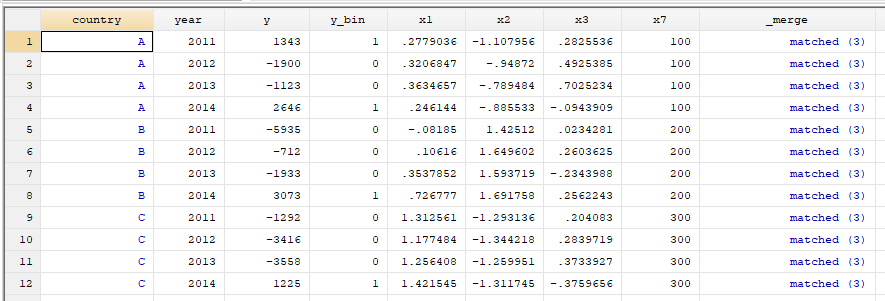Step-by-step procedure for your own data:

• When working on your own data, after the use command, write the path name of the location of your master dataset (in my case, mydata1 is located at "C:/Users/ma3482/Documents/Merge-Append/mydata1.dta"). So, I used the following codes:

use "C:/Users/ma3482/Documents/Merge-Append/mydata1.dta"

• Identify the common variables in your dataset. In my case, country is the common variable. Make sure that after the using command, you are writing the path name of your using dataset's location (in my case, mydata3 is located at "C:/Users/ma3482/Documents/Merge-Append/mydata2.dta"). I used the following codes:

merge m:1 country using "C:/Users/ma3482/Documents/Merge-Append/mydata3.dta"

3. One-to-Many Merge

One-to-many merge is similar to many-to-one merge, except that now the common variable(s) identifies unique observations in the master dataset. In this case, mydata3 (see above) would be considered as master data and mydata1 (see above) as using data. The Stata command for one-to-many merge is  merge 1:m

4. Many-to-Many Merge

We use m:m merge when the common variable(s) does not uniquely identify the observations in either dataset. The use of m:m merge is not usually encouraged. The Stata command for many-to-many merge is  merge m:m

More Notes on Merge:

- Sometimes you will find that a variable with the same name is present in both the master and using datasets. For example, the variable x4 may be there in both the master and using datasets. In this situation, by default, Stata keeps the observations for this duplicate variable from the master dataset and drops the observations from the using dataset. However, you can apply your own choice by adding update or  update replace  after the main command.

• If you use ,update after the main command, missing values in the master dataset for the duplicate variable will be replaced by  values from the using dataset. An example of this code is as follows:

merge 1:1 country year using mydata2, update

• If you use ,update replace after the main command, all values in the master dataset for the duplicate variable will be replaced by values from the using dataset. An example of this code is as follows:

merge 1:1 country year using mydata2, update replace

- While merging datasets, you may sometimes get the following error message from Stata:  "variable id does not uniquely identify observations in the master data" . You may see this message for several reasons, such as

-  using 1:m instead of m:1, or vice-versa.

-  specifying just one common variable when you need two.

- presence of duplicate IDs in your datasets.

To avoid getting this message, inspect datasets carefully before running the merge codes.

For more details about merge, type help merge in Stata, or consult the document, which I have used in preparing this guide, available here: https://www.stata.com/manuals/dmerge.pdf

## Append

- We use append to add observations to a dataset.

- Appending two datasets requires that both datasets have variables with exactly the same name.

- When using categorical data, make sure the categories of variables on both datasets refer to exactly the same thing (e.g., both datasets should have "White" =1,  "Black" = 2, “Hispanic” = 3 ).

Let us append the following two datasets using Stata.

mydata4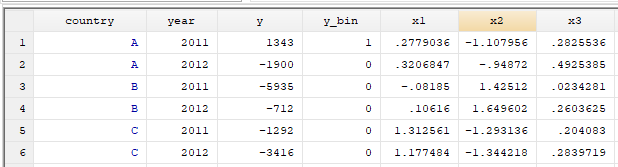mydata5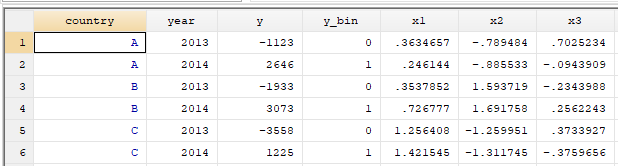Notice the difference between the two datasets. mydata4 contains information for the years 2011 and 2012 for countries A, B, C, and for the variables y, y_bin, x1, x2, and x3. On the other hand, mydata5 contains information for the years 2013 and 2014 for the same countries and variables recorded in mydata4. Basically, we will update the dataset mydata4 by adding observations for all variables for the years 2013 and 2014. Use the following Stata commands to do that.

use https://dss.princeton.edu/training/mydata4.dta
append using https://dss.princeton.edu/training/mydata5.dta

The resulting dataset is as follows.

appended data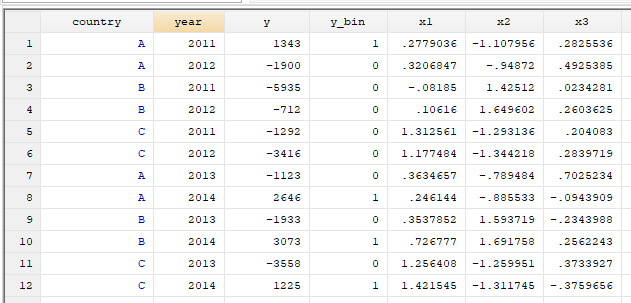You can sort the appended dataset, which will help you inspect your data easily. For sorting, type:

sort country year

The sorted dataset is as follows.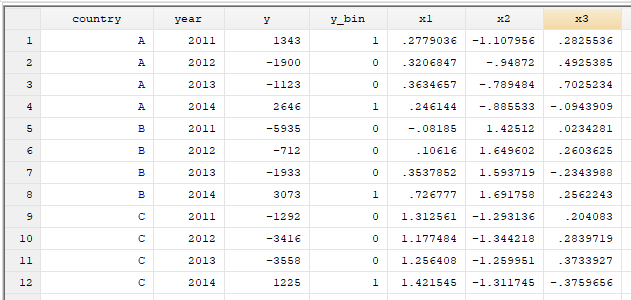Step-by-step procedure for your own data:

• When working on your own data, after the use command, write the path name of the location of your master dataset (in my case, mydata4 is located at "C:/Users/ma3482/Documents/Merge-Append/mydata4.dta"). So, I used the following codes:

use "C:/Users/ma3482/Documents/Merge-Append/mydata4.dta"

• Make sure that after the using command, you are writing the path name of your using dataset's location (in my case, mydata5 is located at "C:/Users/ma3482/Documents/Merge-Append/mydata5.dta"). I used the following codes:

append using "C:/Users/ma3482/Documents/Merge-Append/mydata5.dta"

• For sorting, type:

sort country year

Example of appended data when one dataset is missing one variable

Let us append mydata4 and mydata6.

mydata4mydata6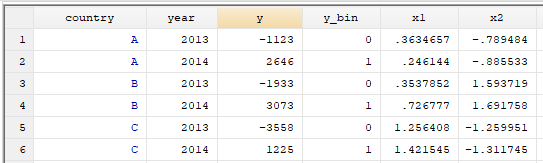Notice that mydata6 does not contain variable x3.

For appending mydata4 and mydata6, use the following Stata codes.

use https://dss.princeton.edu/training/mydata4.dta
append using https://dss.princeton.edu/training/mydata6.dta

appended dataset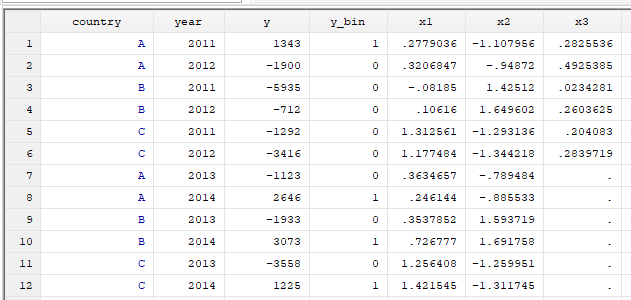Notice the missing observations in the above appended dataset.

## References

Baum, C. F. (2011). Introduction to Stata. Faculty Micro Resource Center, Boston College. Available ate: http://fmwww.bc.edu/GStat/docs/StataIntro.pdfaccessed August, 23 (2022).

DSS Online Training Section https://dss.princeton.edu/training/

Princeton DSS Libguides https://libguides.princeton.edu/dss

STATA FAQ Website http://stata.com/support/faqs/

Stata Manual for Merge https://www.stata.com/manuals/dmerge.pdf

UCLA Resources https://stats.oarc.ucla.edu/stata/